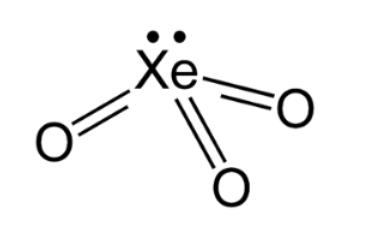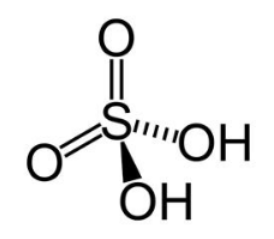QuestionAnswers

# Draw the structure of the following:( i ) ${\rm{Xe}}{{\rm{O}}_{\rm{3}}}$( ii ) ${{\rm{H}}_{\rm{2}}}{\rm{S}}{{\rm{O}}_{\rm{4}}}$

Hint: For hybridisation determination, use the following formula:
${\rm{Hybridisation = }}\dfrac{{{\rm{ }}\begin{array}{*{20}{c}} {{\rm{ Number of valence electrons }}}\\ {{\rm{ on sulphur }}} \end{array}{\rm{ + }}\begin{array}{*{20}{c}} {{\rm{number of monovalent }}}\\ {{\rm{groups attached }}} \end{array} - \begin{array}{*{20}{c}} {{\rm{ charge }}}\\ {{\rm{ with sign }}} \end{array}{\rm{ }}}}{2}$

( i ) ${\rm{Xe}}{{\rm{O}}_{\rm{3}}}$
The central xenon atom has 8 valence electrons. The molecule is electrically neutral with 0 overall charge. Determine the type of the hybridisation:
$\begin{array}{l} {\rm{Hybridisation = }}\dfrac{{{\rm{ }}\begin{array}{*{20}{c}} {{\rm{ Number of valence electrons }}}\\ {{\rm{ on xenon }}} \end{array}{\rm{ + }}\begin{array}{*{20}{c}} {{\rm{number of monovalent }}}\\ {{\rm{groups attached }}} \end{array} - \begin{array}{*{20}{c}} {{\rm{ charge }}}\\ {{\rm{ with sign }}} \end{array}{\rm{ }}}}{2}\\ {\rm{Hybridisation = }}\dfrac{{8 + 0 - 0}}{2}\\ {\rm{Hybridisation = }}\dfrac{8}{2}\\ {\rm{Hybridisation = }}4{\rm{ }} \end{array}$

Here the number of monovalent groups is taken as zero as oxygen is a bivalent group.
Hybridisation =4 suggests ${\rm{s}}{{\rm{p}}^3}$ hybridisation. Three bonding domains and one lone pair of electrons are present around the central xenon atom. The electron pair geometry is tetrahedral and the molecular geometry is trigonal planar. The structure is as shown below:( ii ) ${{\rm{H}}_{\rm{2}}}{\rm{S}}{{\rm{O}}_{\rm{4}}}$
The central sulphur atom has 6 valence electrons. The molecule is electrically neutral with 0 overall charge. Determine the type of the hybridisation:
$\begin{array}{l} {\rm{Hybridisation = }}\dfrac{{{\rm{ }}\begin{array}{*{20}{c}} {{\rm{ Number of valence electrons }}}\\ {{\rm{ on sulphur }}} \end{array}{\rm{ + }}\begin{array}{*{20}{c}} {{\rm{number of monovalent }}}\\ {{\rm{groups attached }}} \end{array} - \begin{array}{*{20}{c}} {{\rm{ charge }}}\\ {{\rm{ with sign }}} \end{array}{\rm{ }}}}{2}\\ {\rm{Hybridisation = }}\dfrac{{6 + 2 - 0}}{2}\\ {\rm{Hybridisation = }}\dfrac{8}{2}\\ {\rm{Hybridisation = }}4{\rm{ }} \end{array}$
Here the number of monovalent groups is taken as two as two hydroxyl groups are monovalent groups and oxygen (having double bond) is bivalent group.
Hybridisation =4 suggests ${\rm{s}}{{\rm{p}}^3}$ hybridisation. Four bonding domains and zero lone pairs of electrons are present around the central sulphur atom. The geometry is tetrahedral. The structure is as shown below:Note:
Hybridisation is the process of mixing two or more atomic orbitals, having almost the same energy, to form the same number of identical and degenerate new types of orbitals. The new orbitals formed are called hybrid orbitals. Hybridisation is used in organic and inorganic chemistry. Hybridisation is a simple theoretical model used to explain molecular geometry. The theory of hybridisation was needed as the original valence bond theory could not explain the molecular geometry.